§ 5 二次曲线

一、圆

[圆的方程、圆心与半径]

 方 程 与 图 形 圆 心 与 半 径 x2 + y2 = R2 或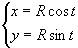(参数方程，t为动径OM与x轴正方向的夹角)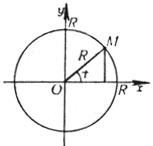圆心 G(0,0) 半径 r = R (x - a)2+(y - b)2 = R2 或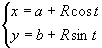(参数方程，t为动径OM与x轴正方向的夹角)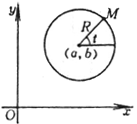圆心 G(a, b) 半径 r = R x2 +y2 +2mx + 2ny + q = 0 m2 + n2 > q r2+2r(mcost + nsint) + q = 0 (极坐标方程)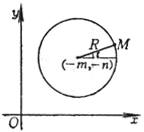圆心 G(-m,- n) 半径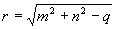r2-2rr0cos(j- j0)+r02 = R2 (极坐标方程)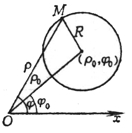圆心 G(r0,j0) 半径 r = R x2 + y2 = 2Rx 或 r = 2Rcosj (极坐标方程)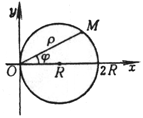圆心 G(R, 0) 半径 r = R x2 + y2 = 2Ry 或 r = 2Rsinj (极坐标方程)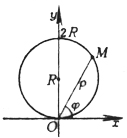圆心 G(0,R) 半径 r = R

[圆的切线]

x2 + y2 = R2 上一点M(x0, y0)的切线方程为

x0x + y0y = R2

x2 + y2 + 2mx + 2ny + q = 0 上一点M(x0, y0)的切线方程为

x0x + y0y + m(x + x0) + n(y + y0) + q = 0

[两个圆的交角、圆束与根轴]

 方 程 与 图 形 公 式 与 说 明 两个圆的交角 C1 x2 +y2 +2m1x +2n1y +q1 = 0 C2 x2 +y2 +2m2x +2n2y +q2 = 0 两个圆的交角是指它们在交点的两条切线的夹角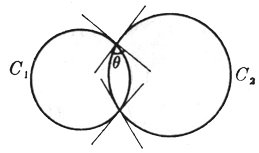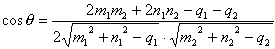式中q 表示两个圆C1和C2的交角，因为公式中不包含交点的坐标，所以在两交点的两交角必相等. 两个圆C1和C2正交条件为 2m1m2 + 2n1n2 - q1 - q2 = 0 圆束× 两个圆的根轴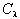C1 + l C2 = 0 (l 为参数) 或 (l+1)(x2+y2) +2(m1+l m2)x +(n1+ln2)y + (q1 +lq2) = 0 根轴方程为 2(m1 - m2)x + 2(n1 - n2)y + (q1 - q2) = 0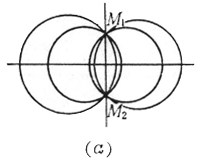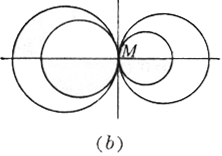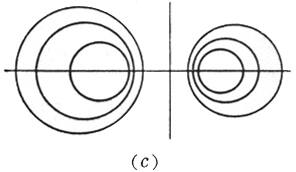对l (l ¹ -1)的一个确定值，表示一个圆.当l 取一切值(l ¹ -1)时，所表示的圆的全体，称为圆束.l = -1时，为一直线，称为两个圆C1和C2的根轴.根轴与C1和C2的连心线垂直，束中任一圆的圆心在C1和C2的连心线上，且分连心线的比等于l . (a)如果C1和C2 相交于两点M1，M2，则束中一切圆都通过两交点M1，M2，它们的根轴就是它们的公共弦.这时圆束称为共轴圆系(图(a)). (b)如果C1和C2切于一点M，则束中一切圆都在一点M相切，根轴就是在点M的公切线(图(b)). (c)如果C1和C2不相交，则束中一切圆都不相交，根轴也与圆束中一切圆都不相交(图(c)). 从点P作两个圆C1和C2的切线，具有相等切线长的点P的轨迹就是根轴.两个同心圆的根轴是从公共圆心到无穷远处的直线.三个圆中每对圆的根轴(共三个)交于一点，它称为根心.若三个圆心共线，则其根心在无穷远处.

[反演] C为一定圆，O为圆心，r为半径(7.1)，对平面上任一点M，有一点M¢ 与它对应.使得满足下列两个条件：

iO, M, M¢ 共线，

iiOM× OM¢ = r2

O为原点，则一切反演点M(x, y)M¢ (x¢ ,y¢ )的对应方程为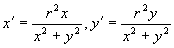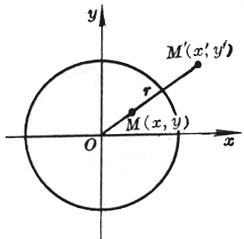1° 不通过反演中心的一条直线变为通过反演中心的一个圆.

2° 通过反演中心的圆变为不通过反演中心的直线.

3° 通过反演中心的一条直线变为它自己.

4° 不通过反演中心的圆变为不通过反演中心的圆.

5° 反演圆变为它自己.

6° 与反演圆正交的圆变为它自己，其逆也真.

7° 如果两条曲线C1C2交于一点M，则经过反演后的曲线C1¢ , C2¢ 必交于M的反演点M¢ .

8° 如果两条曲线C1, C2在一点M相切，则经过反演后的曲线C1¢ , C2¢ 必在M的反演点M¢ 相切.

9° 两条曲线的交角在反演下是不变的.由此可见，反演是一个保角变换.